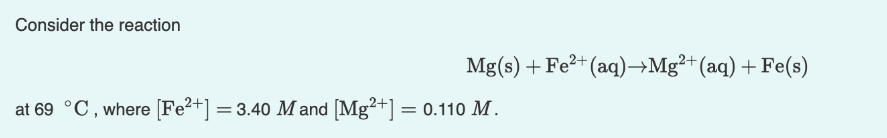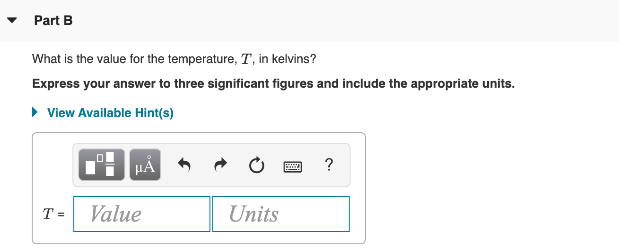# Consider the reaction Mg(s) + Fe^2+ (aq) → Mg^2+(aq) + Fe(s) at 69 °C, where [Fe^2+] = 3.40 M and [Mg^2+] = 0.110 M. What is the value for the temperature, T, in kelvins? Express your answer to three significant figures and include the appropriate units.